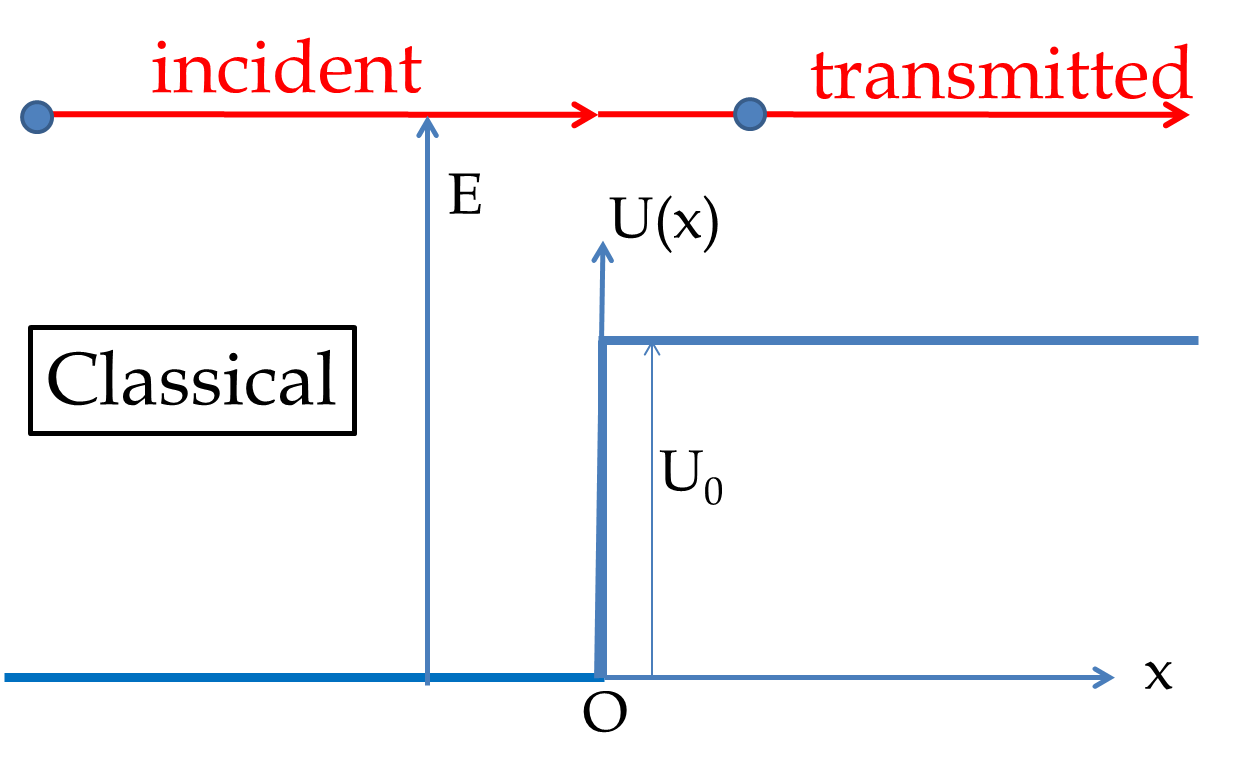## Section54.6Reflection of Wave

In classical world, if a plane flies over a mountain, the plane is not reflected by the mountain. But, in quantum world, if you send a bunch of “planes”, some of them will be reflected back. That is, each plane has a probablity of reflecting back even when it has enough energy to fly over the mountain. In this section we will see this in a simple example shown in Figure 54.6.1.Figure 54.6.1. The reflection of a particle from a finite potential step when the energy of the particle is greater than the energy of the potential step. Classically, the particle should go over the step and continue on its original path. In quantum mechanics, there is a probability that the particle will be reflected back!

If energy $E$ of the particle is more than the potential energy of the barrier $U_0\text{,}$ then in the classical world of everyday life such as plane flying over a mountain, the particle will just continue onward. But in quantum mechanics, the wave function in the $x \lt 0$ region will be a superposition of a wave moving towards the positive $x$-axis, $\psi_\text{in}\text{,}$ and another wave moving towards the negative $x$-axis, $\psi_\text{re}\text{.}$

\begin{equation*} \psi_I = \psi_\text{in} + \psi_\text{re}. \end{equation*}

In the region $x>0$ we have one wave - the transimtted wave moving towards the positive $x$-axis, $\psi_\text{tr}\text{.}$

\begin{equation*} \psi_{II} = \psi_\text{tr}. \end{equation*}

To write the solutions it is better to introduce the following constants.

\begin{equation*} k = \dfrac{\sqrt{2mE}}{\hbar},\quad k' = \dfrac{\sqrt{2m(E-U_0)}}{\hbar}, \quad \omega = \dfrac{E}{\hbar}. \end{equation*}

In terms of these constants the incoming, reflected and transmitted waves are:

\begin{equation*} \psi_\text{in} = A e^{ikx-i\omega t},\quad \psi_\text{re} = B e^{-ikx -i\omega t}, \quad \psi_\text{tr} = C e^{ikx-i\omega t}, \end{equation*}

where $A\text{,}$ $B\text{,}$ and $C$ are the amplitudes of the incoming wave, the reflected wave, and the transmitted wave. The wave function in the two regions are:

\begin{align*} \amp \psi_I(x,t) = A e^{ikx-i\omega t} + B e^{-ikx -i\omega t}.\\ \amp \psi_{II}(x,t) = C e^{ikx-i\omega t}. \end{align*}

The wave functions in the two regions join at $x=0$ such that they have same amplitudes and same slopes with respect to $x$ at $x=0\text{.}$ These are called boundary conditions on the wave function.

\begin{align} \amp \psi_I(0,t) = \psi_{II}(0,t) \longrightarrow A + B = C.\label{eq-reflect-potential-1}\tag{54.6.1}\\ \amp \left.\dfrac{d\psi_I}{dx}\right|_{x=0} = \left.\dfrac{d\psi_{II}}{dx}\right|_{x=0} \longrightarrow A - B = \dfrac{k'}{k}C. \label{eq-reflect-potential-2}\tag{54.6.2} \end{align}

Equations (54.6.1) and (54.6.2) can be solved for B/A and C/A as

The probability current of incoming wave tells us the flux of the incoming wave. Suppose there is a source of particles at $x=-\infty\text{,}$ then the number of particles arriving at $x=0$ per unit time will be proportional to $j_\text{in}\text{.}$ In that experiment, the particle leaving $x=0$ towards $x=-\infty$ will be $j_\text{re}$ and towards $x=\infty$ will be $j_\text{tr}\text{.}$ Therefore, the flux of reflected and transmitted waves should add up to the flux of the incoming wave. Let us verify this by computing the probability currents for the three waves.
This gives the desired conservation of probabilities arrivig at $x=0$ and leaving $x=0$ as expected.
TODO: \​begin{exercise} An electron of energy 10 eV is shot towards a region where there is a repulsive potential barrier of 4 eV. Suppose the barrier starts at $x=0$ and continues towards the positive $x$-axis and the electron is shot at the barrier from a far-away place on the negative $x$-axis. (a) Find the wave number $k$ of the electron when it is in region $x\lt 0$. (b) Find the wave number $k$ of the electron when it is in region $x>0$. (c) What is the probability that the electron will be reflected? (c) What is the probability that the electron will be transmitted? \end{exercise} \​begin{exercise} A proton of energy 10 MeV is shot towards a region where there is a repulsive potential barrier of 4 MeV. Suppose the barrier starts at $x=0$ and continues towards the positive $x$-axis and the proton is shot at the barrier from a far-away place on the negative $x$-axis. (a) Find the wave number $k$ of the proton when it is in region $x \lt 0$. (b) Find the wave number $k$ of the proton when it is in region $x>0$. (c) What is the probability that the proton will be reflected? (c) What is the probability that the proton will be transmitted? \end{exercise} \​begin{exercise} Electrons of energy 20 eV are shot towards a region where there is a repulsive potential barrier of energy less than 20 eV. Suppose the barrier starts at $x=0$ and continues towards the positive $x$-axis and the electron is shot at the barrier from a far-away place on the negative $x$-axis. It is found that 25\% of the electrons continue on towards positive $x$-axis and 75\% of the electrons are reflected back. What is energy of the barrier? \end{exercise} \​begin{exercise} Electrons of unknown energy are shot towards a region where there is a repulsive potential barrier of energy 100 eV. Suppose the barrier starts at $x=0$ and continues towards the positive $x$-axis and the electron is shot at the barrier from a far-away place on the negative $x$-axis. It is found that 25\% of the electrons continue on towards positive $x$-axis and 75\% of the electrons are reflected back. What is energy of the electrons? \end{exercise} \​begin{exercise} Electrons of unknown energy are shot towards a region where there is a repulsive potential barrier of energy 100 eV. Suppose the barrier starts at $x=0$ and continues towards the positive $x$-axis and the electron is shot at the barrier from a far-away place on the negative $x$-axis. It is found that 25\% of the electrons continue on towards positive $x$-axis and 75\% of the electrons are reflected back. We wish to increase the percentage of electrons transmitted in the region of the barrier to 50\%. By what factor should the energy of the electrons be increased? \end{exercise}